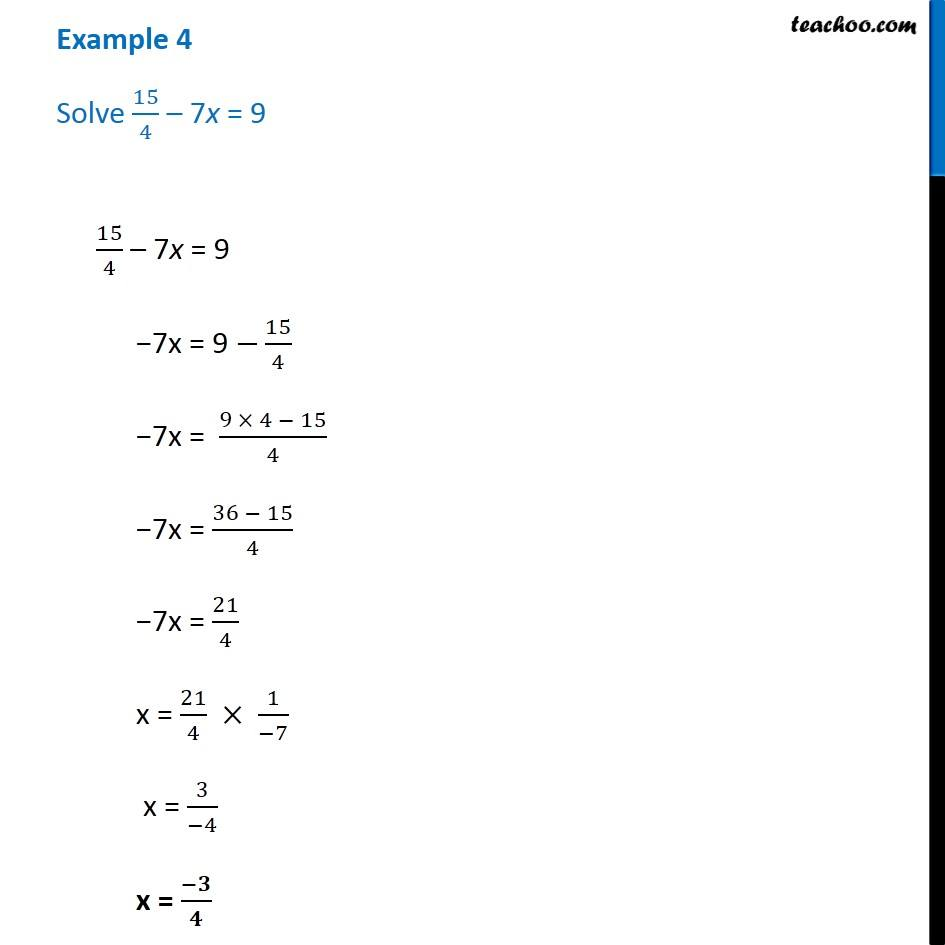Examples

Chapter 2 Class 8 Linear Equations in One Variable
Serial order wiseLearn in your speed, with individual attention - Teachoo Maths 1-on-1 Class

### Transcript

Question 4 - Chapter 2 Class 8 - Linear Equations in one variable - NCERT Book Solve 15/4 – 7x = 9 15/4 – 7x = 9 −7x = 9 −15/4 −7x = (9 × 4 − 15)/4 −7x = (36 − 15)/4 −7x = 21/4 x = 21/4 × 1/(−7) = 3/(−4) x = -3/4